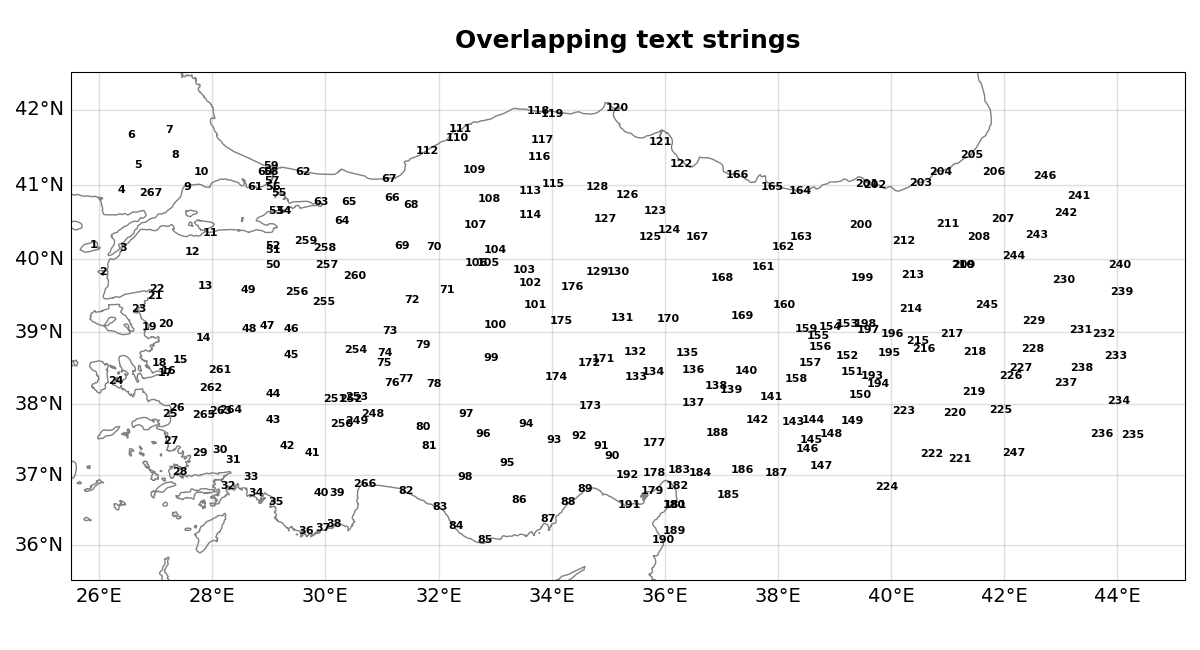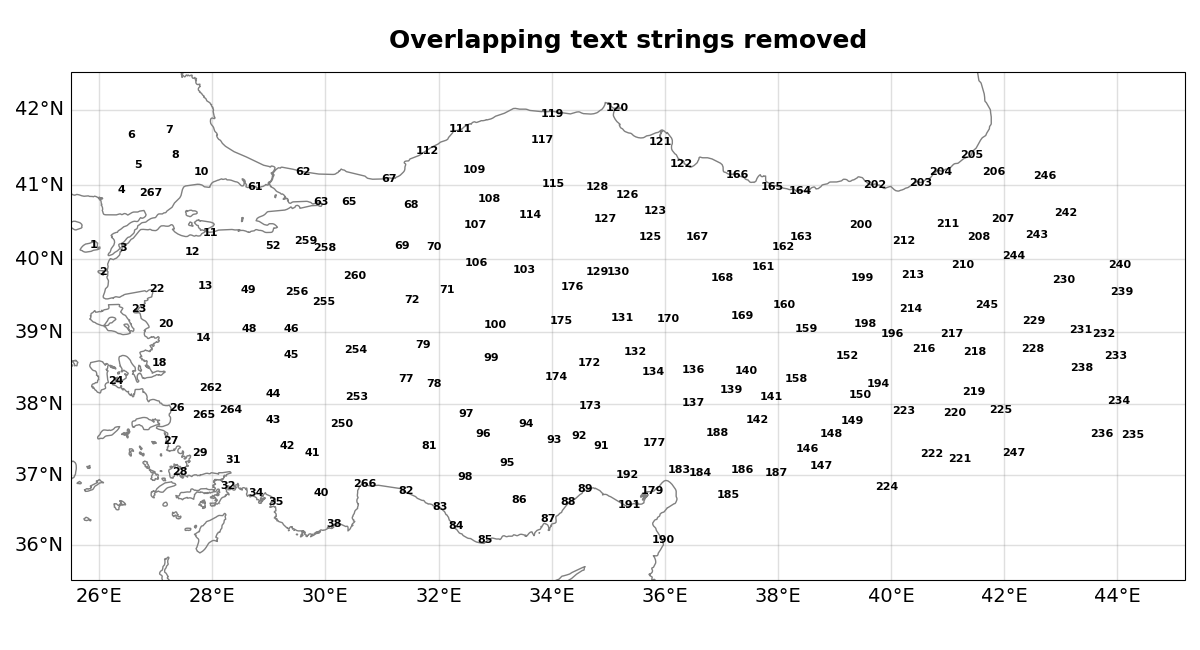# NCL_station_3.py#

This script illustrates the following concepts:
• Drawing station numbers on a map, and removing ones that overlap

• Attaching lots of text strings to a map

• Using Cartopy’s GeoAxes.gridlines as a workaround to adding tick labels on Axes with Mercator (or another) map projection

See following URLs to see the reproduced NCL plot & script:

Import packages:

```import numpy as np
import pandas as pd
import cartopy.crs as ccrs
import cartopy.feature as cfeature
from matplotlib import pyplot as plt
import matplotlib.ticker as mticker

import geocat.datafiles as gdf
```

```# Open a ascii data file using pandas read_csv and assigning column names
gdf.get('ascii_files/istasyontablosu_son.txt'),
delimiter='\\s+',
names=['index', 'station', 'year1', 'year2', 'number', 'lat', 'lon'])

# Get number of stations
npts = len(ds)

# Extract variables
no = ds.index + 1  # +1 because Pandas' RangeIndex defaults start with 0
lat = ds.lat
lon = ds.lon
```

Helper function to add plot elements to the axes

```def create_axes(maintitle):

# Generate figure (set its size (width, height) in inches)
fig = plt.figure(figsize=(12, 6.5))

# Generate axes
ax = plt.axes(projection=ccrs.Mercator())

# Set extent to show particular area of the map
ax.set_extent([25.5, 45.2, 35.5, 42.5], ccrs.PlateCarree())

# Add state boundaries other lake features

# Draw gridlines
gl = ax.gridlines(crs=ccrs.PlateCarree(),
draw_labels=True,
dms=False,
x_inline=False,
y_inline=False,
linewidth=1,
color="gray",
alpha=0.25)

# Set frequency of gridlines in the x and y directions
gl.xlocator = mticker.FixedLocator(np.arange(26, 45, 2))
gl.ylocator = mticker.FixedLocator(np.arange(36, 43, 1))

# Turn top/right labels
gl.top_labels = False
gl.right_labels = False

# Set label sizes
gl.xlabel_style = {"rotation": 0, "size": 14}
gl.ylabel_style = {"rotation": 0, "size": 14}

# Manually turn off ticks on top and right spines
ax.tick_params(axis='x', top=False)
ax.tick_params(axis='y', right=False)

ax.set_title(maintitle, fontweight='bold', fontsize=18, y=1.03)

return fig, ax
```

Plot with texts overlapping

```fig, ax = create_axes('Overlapping text strings')

# Add all station number texts
for i in range(npts):
ax.text(lon[i],
lat[i],
no[i],
fontsize=8,
fontweight='bold',
va='center',
ha='center',
transform=ccrs.PlateCarree())

# Show the plot
plt.tight_layout()
plt.show()
```Plot without texts overlapping

```fig, ax = create_axes('Overlapping text strings removed')

# Transpose the array of longitude and latitude for easier access of the location of each station point
location = np.transpose(np.array([lon, lat]))

# Create an array of booleans denoting if station would be removed
remove = np.full(npts, False)

# Currently minimum distance is calculated through finding distance between two suitable stations
# In the future we would like to find mindist by finding the width and height of texts in pixel coordinates
mindist = np.sqrt(np.sum(np.square(location - location)))

# Tag station to be removed using array `remove`
# Loop through every pair of stations and calculate distances between them
for i in range(npts):
for j in range(npts):
# Calculate euclidean distance with numpy functions
dist = np.sqrt(np.sum(np.square(location[j] - location[i])))
if dist <= mindist and i != j and not remove[j]:
# Tag one of the stations to be removed if distance between them allows for overlap,
# they are different stations, and if the other station will not be removed
remove[i] = True

# Add text if it is not tagged to be removed
for i in range(npts):
if not remove[i]:
ax.text(lon[i],
lat[i],
no[i],
fontsize=8,
fontweight='bold',
va='center',
ha='center',
transform=ccrs.PlateCarree())

# Show the plot
plt.tight_layout()
plt.show()
```Total running time of the script: ( 0 minutes 11.126 seconds)

Gallery generated by Sphinx-Gallery